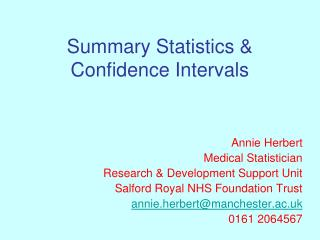DownloadDownload PresentationSummary Statistics & Confidence Intervals

# Summary Statistics & Confidence Intervals

Télécharger la présentation## Summary Statistics & Confidence Intervals

- - - - - - - - - - - - - - - - - - - - - - - - - - - E N D - - - - - - - - - - - - - - - - - - - - - - - - - - -
##### Presentation Transcript

1. Summary Statistics & Confidence Intervals Annie Herbert Medical Statistician Research & Development Support Unit Salford Royal NHS Foundation Trust annie.herbert@manchester.ac.uk 0161 2064567

2. Timetable

3. Outline • Sampling • Summary statistics • Confidence intervals • Statistics Packages

4. ‘Population’ and ‘Sample’ • Studying population of interest. Usually would like to know typical value and spread of outcome measure in population. • Data from entire population usually impossible or inefficient/expensive so take a sample (even census data can have missing values). • Sample must be representative of population. • Randomise!

5. E.g. Randomised Controlled Trial (RCT) OUTCOME GROUP 1 POPULATION SAMPLE RANDOMISATION GROUP 2 OUTCOME

6. Types of Data • Numerical/Continuous • Example: • Weight • Pain Score • Graphs: • Histogram • Box and Whisker Plot • Summary: • Mean & Standard Deviation (SD) • Median & Inter-quartile range (IQR) Categorical Example: • Yes/No • Blood Group Graphs: • Bar Chart • Pie Chart Summary: • Frequency (n) • Proportion (%)

7. Types of Average(‘Average’ - a number which typifies a set of numbers) • Mean =Total divided by n • Median =Middle value • Mode =Most common value/group (rarely used)

8. Types of Average - Example Pain score data: 10, 8, 7, 7, 1, 7, 6, 5, 3, 4 Ordered: 1, 3, 4, 5, 6, 7, 7, 7, 8, 10 Mean = (1 + 3 + 4 + … + 10) ÷ 10 = 5.8 Median = (6+7) ÷ 2 = 6.5 Mode = 7 Median 2nd 3rd 5th 6th 8th 9th

9. Mean or Median? • Roughly Normally distributed: • Mean or median • Mean by convention • Skewed: • Median • Less affected by extreme values

10. Variation and Spread • Standard Deviation (‘SD’) - Average distance from mean - Use alongside mean • Inter-Quartile Range (‘IQR’) - Range in which middle 50% of the data lie (middle 50% when ordered) - Use alongside median • Range - Highest and lowest value - Possibly quote in addition to SD/IQR

11. Types of Variation - Example Pain score data: 10, 8, 7, 7, 1, 7, 6, 5, 3, 4 Ordered: 1, 3, 4, 5, 6, 7, 7, 7, 8, 10 SD = 2.6 IQR = (3.75, 7.25) Range = (1,10) Median 2nd 3rd 5th 6th 8th 9th IQR

12. Standard Error • Not the same as standard deviation. • Calculated using a measure of variability and sample size. • Used to construct confidence intervals. • Not very informative when given alongside statistics or as error bars on a plot.

13. Sample statistic is the best guess of the (true) population value • E.g. Sample mean is the best estimate of mean in population. • Mean likely to be different if take a new sample from the population. • Know that estimate not likely to be exactly right.

14. Confidence Intervals (CIs) • Confidence interval = “range of values that we can be confident will contain the true value of the population”. • The “give or take a bit” for best estimate. • Convention is to use a 95% confidence interval (‘95% CI’). • But also leaves 5% confidence that this interval does not contain the true value.

15. Example: Legislation for smoke-free workplaces and health of bar workers in Ireland: before and after study (Allwright et al; BMJ Oct 2005)

16. Example: Supplementary feeding with either ready-to-use fortified spread or corn-soy blend in wasted adults starting antiretroviral therapy in Malawi (MacDonald et al; BMJ May 2009) “After 14 weeks, patients receiving fortified spread had a greater increase in BMI and fat-free body mass than those receiving corn-soy blend: 2.2 (SD 1.9) v 1.7 (SD 1.6) (difference 0.5, 95% confidence interval 0.2 to 0.8), and 2.9 (SD 3.2) v 2.2 (SD 3.0) kg (difference 0.7 kg, 0.2 to 1.2 kg), respectively.”

17. Example: Sample size matters What proportion of patients attending clinic are satisfied?

18. Example: % confidence matters What proportion of patients attending clinic are satisfied?

19. p-values vs. Confidence Intervals • p-value: • Weight of evidence to reject null hypothesis • No clinical interpretation • Confidence Interval: • Can be used to reject null hypothesis • Clinical interpretation • Effect size • Direction of effect • Precision of population estimate

20. So… it’s not all about p-values! • For some hypotheses p-value and CI will both indicate whether to reject it or not. • A CI will also provide an estimate, as well as a range for that estimate. • General medical journals prefer CI.

21. Statistical Packages

22. Thanks for listening!